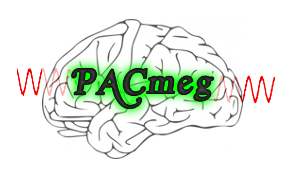PACmeg

MATLAB scripts for detecting and validating phase amplitude coupling (PAC) in electrophysiological data.

Written and maintained by Robert Seymour, June 2017 - October 2019.Manuscript & Citation

If you use these scripts, please cite:

Seymour, R. A., Rippon, G., & Kessler, K. (2017).
The Detection of Phase Amplitude Coupling During Sensory Processing.
Frontiers in Neuroscience 11, 487.
https://doi.org/10.3389/fnins.2017.00487

PAC Function

The pacMEG.m function can be used to create a phase x amplitude comodulogram, for data arranged in trial x time matrix:

%%%%%%%%%%%%%%%%%%%%%%%%%%%%%%%%%%%%%%%%%%%%%%%%%%%%%%%%%%%%%%%%%%%%%%%%%%%%%%%%%%%
% PACmeg: a function to do PAC
%
% Author: Robert Seymour ([email protected])
%
%%%%%%%%%%%
% Inputs:
%%%%%%%%%%%
%
% data              = data for PAC (size: trials*time)
% cfg.Fs            = Sampling frequency (in Hz)
% cfg.phase_freqs   = Phase Frequencies in Hz (e.g. [8:1:13])
% cfg.amp_freqs     = Amplitude Frequencies in Hz (e.g. [40:2:100])
% cfg.filt_order    = Filter order used by ft_preproc_bandpassfilter
%
% amp_bandw_method  = Method for calculating bandwidth to filter the
%                   ampltitude signal:
%                        - 'number': +- nHz either side
%                        - 'maxphase': 1.5*max(phase_freq)
%                        - 'centre_freq': +-2.5*amp_freq
% amp_bandw         = Bandwidth when cfg.amp_bandw_method = 'number';
%
% cfg.method        = Method for PAC Computation:
%                   ('Tort','Ozkurt','PLV','Canolty)
%
% cfg.surr_method   = Method to compute surrogates:
%                        - '[]': No surrogates
%                        - 'swap_blocks': cuts each trial's amplitude at
%                        a random point and swaps the order around
%                        - 'swap_trials': permutes phase and amp from
%                        different trials
% cfg.surr_N        = Number of iterations used for surrogate analysis
%
% cfg.mask          = filters ALL data but masks between times [a b]
%                   (e.g. cfg.mask = [100 800]; will
%
% cfg.avg_PAC       = Average PAC over trials ('yes' or 'no')
%
%%%%%%%%%%%
% Outputs:
%%%%%%%%%%%
%
% - MI_matrix_raw   = comodulagram matrix (size: amp*phase)
% - MI_matrix_surr  = surrogate comodulagram matrix (size: surr*amp*phase)
%
%%%%%%%%%%%%%%%%%%%%%%%%%%%%%%%%%%%%%%%%%%%%%%%%%%%%%%%%%%%%%%%%%%%%%%%%%%%%%%%%%%%

Currently the function only accepts data from a single time-series which could be obtained using an atlas-based approach (e.g. AAL atlas or HCP-MMP 1.0).

Example use:

% To produce a PAC comodulogram using the
% Tort et al., (2010) approach, between 2-10Hz phase and
% 30-100Hz amplitude, with 200 surrogates:

cfg                     = [];
cfg.Fs                  = 1000;
cfg.phase_freqs         = [2:1:10];
cfg.amp_freqs           = [30:2:100];
cfg.method              = 'tort';
cfg.filt_order          = 3;
cfg.surr_method         = 'swap_blocks';
cfg.surr_N              = 200;
cfg.amp_bandw_method    = 'number';
cfg.amp_bandw           = 16;

[MI_raw,MI_surr]        = PACmeg(cfg,signal);

NOTE:

Please be aware of the various methodological pitfalls in PAC analysis before applying the scripts to your own data (see Seymour, Kessler & Rippon manuscript).

For more information/queries please raise an ISSUE within Github or email me: [email protected]. I am also very keen for collaborations to help improve and expand this code.

Data Sharing (Seymour et al., 2017)

The data discussed in Seymour et al., (2017) was shared opnely usiing Figshare.

Scripts can be easily adapted for your computer by modifying the sensory_PAC.m script:

data_dir = 'path_to_data';
scripts_dir = 'path_to_scripts';
fieldtrip_dir = 'path_to_fieldtrip';
subject = {'sub-01','sub-02','sub-03','sub-04','sub-05','sub-06','sub-07',...
'sub-08','sub-09','sub-10','sub-11','sub-12','sub-13','sub-14',...
'sub-15','sub-16'};

Fieldtrip version 20161024 was used during data analysis, and can be found from the /fieldtrip folder.

Analysis for reproducing Seymour et al., (2017)

• 1_preprocessing_elektra_frontiers_PAC.m = this script performs simple preprocessing steps and removes bad trials

• 2_get_source_power.m = this script performs source analysis in the gamma (40-60Hz) and alpha (8-13Hz) bands.

• 3_get_VE_frontiers_PAC.m = this script computes a V1 virtual electrode, using the HCP-MMP-1.0 atlas. Other atlases could easy be used (e.g. the AAL atlas).

• 4_calc_pow_change.m = this script calculates the change in oscillatory power (1-100Hz) using the V1 virtual electrode.

• 5_visual_PAC_four_methods.m = this script uses 4 different methods to quantify alpha-gamma PAC. Non-parametric statistics are then applied to determine changes in PAC between baseline and grating periods.

• 6_check_non_sinusoidal.m = this script checks the low-frequency phase for differences in non-sinusoidal oscillations.

• 7_simulated_PAC_analysis.m = this script simulates PAC, checks for the detection of this coupling using three approaches, and investigates how much data is needed for reliable PAC estimates.

Get A Weekly Email With Trending Projects For These Topics
No Spam. Unsubscribe easily at any time.
Matlab (44,918
Time Series (2,021
Eeg (428
Neural (181
Connectivity (133
Meg (74
Oscillations (22
Phase Amplitude Coupling (2
Related Projects